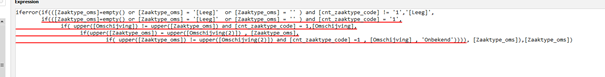# Nested if then else statements

Yesterday I updates easyrmorph from 4.5.2 to 4.6.2
One of my projects isnt working anymore. The problem is a nested if then else statement isnt working anymore.

iferror(if(([Zaaktype_oms]=empty() or [Zaaktype_oms] = ‘[Leeg]’ or [Zaaktype_oms] = ‘’ ) and [cnt_zaaktype_code] != ‘1’,’[Leeg]’,
if(([Zaaktype_oms]=empty() or [Zaaktype_oms] = ‘[Leeg]’ or [Zaaktype_oms] = ‘’ ) and [cnt_zaaktype_code] = ‘1’,
if( upper([Omschijving]) != upper([Zaaktype_oms]) and [cnt_zaaktype_code] = 1,[Omschijving],
if(upper([Zaaktype_oms]) = upper([Omschijving(2)]) , [Zaaktype_oms],
if( upper([Zaaktype_oms]) != upper([Omschijving(2)]) and [cnt_zaaktype_code] =1 , [Omschijving] , ‘Onbekend’)))), [Zaaktype_oms]),[Zaaktype_oms])

I installed the 4.5.2. version again and it is working again.The if() function must have 3 arguments. The old version incorrectly accepted an if() function with 2 or 4 arguments which led to incorrect calculations. It was fixed in the new version. In your expression, the 1st if() has 4 arguments, and the 2nd nested if() function has only 2 arguments which is why the new version shows the error “if can only have 3 arguments”. Probably, it was caused by misplaced 3rd from the end parenthesis.

The correct expression probably should be as follows (check it):

iferror(if(([Zaaktype_oms]=empty() or [Zaaktype_oms] = ‘[Leeg]’ or [Zaaktype_oms] = ‘’ ) and [cnt_zaaktype_code] != ‘1’,’[Leeg]’,
if(([Zaaktype_oms]=empty() or [Zaaktype_oms] = ‘[Leeg]’ or [Zaaktype_oms] = ‘’ ) and [cnt_zaaktype_code] = ‘1’,
if( upper([Omschijving]) != upper([Zaaktype_oms]) and [cnt_zaaktype_code] = 1,[Omschijving],
if(upper([Zaaktype_oms]) = upper([Omschijving(2)]) , [Zaaktype_oms],
if( upper([Zaaktype_oms]) != upper([Omschijving(2)]) and [cnt_zaaktype_code] =1 , [Omschijving] , ‘Onbekend’)))), [Zaaktype_oms]),[Zaaktype_oms])

For better readability, I would highly recommend using the new IF and LET operators, or the “Rule” action. Here is what it would look like rewritten using the new operators:

``````LET no_zt = [Zaaktype_oms]=empty()
LET zt_leeg = [Zaaktype_oms] = '[Leeg]'
LET zt_empty = [Zaaktype_oms] = ''
LET cnt1 = [cnt_zaaktype_code] = '1'
LET zk_O = upper([Omschijving]) = upper([Zaaktype_oms])
LET zk_O2 = upper([Zaaktype_oms]) = upper([Omschijving(2)])

iferror(
IF (no_zt or zt_leeg or zt_empty) and not cnt1
THEN '[Leeg]'
ELSE
IF (no_zt or zt_leeg or zt_empty) and cnt1
THEN
IF not zk_O and cnt1
THEN [Omschijving]
ELSE
IF zk_O2
THEN [Zaaktype_oms]
ELSE
IF not zk_O2 and cnt1
THEN [Omschijving]
ELSE 'Onbekend'
ELSE [Zaaktype_oms]
,[Zaaktype_oms]
)``````